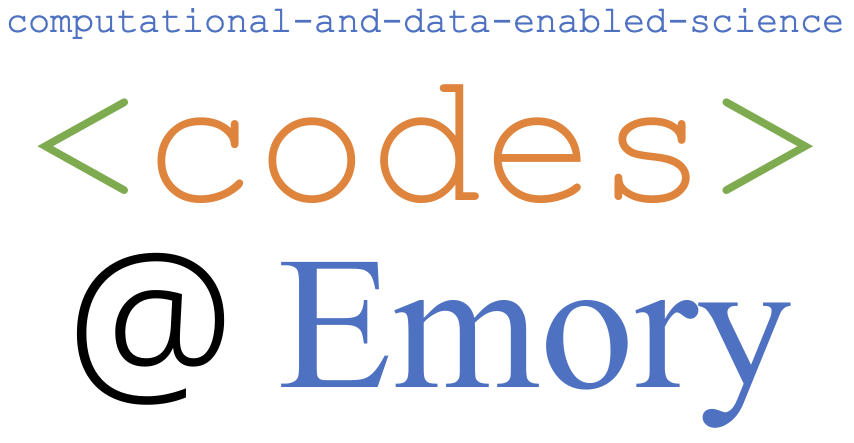Our group highly values teaching and offers a comprehensive set of courses on scientific computing, applied mathematics, data science, mathematical modeling, and its applications. All our courses are taught in relatively small sizes, allowing close interactions with students.

1. MATH 315: Numerical Analysis
2. MATH 345: Mathematical Modeling
3. MATH 347: Introduction to Nonlinear Optimization
4. MATH 351: Partial Differential Equations
5. MATH 352: PDEs in Action
6. MATH 361&362: Probability & Statistics

### Slected Special Topics Undergraduate-Level Courses

1. Progamming for Mathematics of Data Science (Fall 2022)
2. Convex Optimization (Fall 2022)

1. MATH 515: Numerical Analysis I (Numerical Linear Algebra)
2. MATH 516: Numerical Analysis II
3. MATH 517: Numerical Analysis III (Iterative Methods)
4. CS555: Parallel Processing
5. MATH 561: Matrix Analysis
6. MATH 571: Numerical Optimization
7. MATH 572: Numerical Partial Differential Equations

#### Selected Special Topics Graduate-Level Courses

1. RTG Seminar: Computational Methods for Data Science (Fall 2021)
2. Numerical Methods for Deep Learning (Spring 2020)
3. Data Assimilation (Spring 2018)
4. Numerical Methods for Deep Learning (Spring 2018)
5. Structured Matrix Computation (Fall 2017)
6. Bayesian Inverse Problems (Spring 2016)
7. Advanced Numerical Linear Algebra Methods with Applications
8. Fluid Dynamics